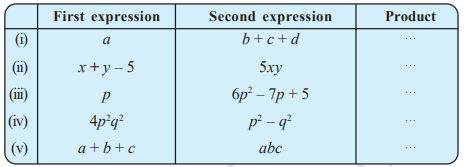# Class 8 Maths Exercise 9.3 Solutions : Algebraic Expressions and Identities

In this page we have Class 8 Maths Exercise 9.3 Solutions for Algebraic Expressions and Identities. This exercise has questions about multiplication of polynomials like mononial to binomial and mononial to Trinomial. Hope you like them and do not forget to like , social share and comment at the end of the page.

## Class 8 Maths Exercise 9.3 Solutions

Question 1
Carry out the multiplication of the expressions in each of the following pairs.
(i) 4p, q + r
(ii) ab, a - b
(iii) a + b, 7a²b²
(iv) a2- 9, 4a
(v) pq + qr + rp, 0
1. 4p(q + r) =  4pq + 4pr
2. ab(a - b) = a2b - ab2
3. (a + b) (7a2b2) = 7a3b2 + 7a2b3
4. (a2 - 9)(4a) = 4a3 - 36a2
5. (pq + qr + rp) x 0 = 0

Question 2
Complete the table.(i) a(b + c + d) = ab + ac + ad

(ii) (x + y – 5) 5xy= 5x2y + 5xy2 -25xy
(iii)p(6p2– 7p + 5)=6p3 -7p2 +5p
(iv) 4p2q2(p2 - q2)=4p4q2 -4p2q4
(v) (a + b + c)abc= a2bc + ab2c + abc2

Question 2
Find the product.
(i) a2 x (2a22) x (4a26)
(ii) (2xy/3) ×(-9x2y2/10)
(iii) (-10pq3/3) ×(6p3q/5)
(iv) x (x2) x (x3) x (x8)
Answer: We will use the below property extensively in above questions
am x an x ao = am+n+o
(i) As you know
So, we get
a2 x (2a22) x (4a26) = 8a48
(ii) (2xy/3) ×(-9x2y2/10)
=(-3x3y3/5)
(iii) (-10pq3/3) ×(6p3q/5)
=(-4p4q4)
(iv)( x) x (x2) x (x3) x (x8)
x14

Question 3
(a) Simplify 3x (4x - 5) + 3 and find its values for (i) x = 3 (ii) x =1/2
(b) Simplify a (a2+ a + 1) + 5 and find its value for (i) a = 0, (ii) a = 1 (iii) a = - 1.
(a)
(i) Putting x=3 in the equation we get
12x2 - 15x + 3 =108-45+3 = 66
(ii) putting x=1/2 in the equation we get
$12 \times \frac {1}{2} - \frac {15}{2} + 3 =3 - \frac {15}{2} -3=\frac {-3}{2}$
(b)
a(a2a+1)
=a3+a2+a
(i) putting a= 0 in the equation we get
03+02+0=0
(ii) putting a=1 in the equation we get
1+ 1+ 1 = 1 + 1 + 1 = 3
(iii) Putting a = -1 in the equation we get
-13+1-1 = -1 + 1 + 1 = 1

Question 5
(a) Add: p ( p - q), q ( q - r) and r ( r - p)
(b) Add: 2x (z - x - y) and 2y (z - y - x)
(c) Subtract: 3l (l - 4 m + 5 n) from 4l ( 10 n - 3 m + 2 l )
(d) Subtract: 3a (a + b + c ) - 2 b (a - b + c) from 4c ( - a + b + c )
Answer: (a) (p2 - pq) + (q2 - qr) + (r2 - pr)
= p2 + q2 + r2 - pq - qr - pr
(b) (2xz - 2x2 - 2xy) + (2yz - 2y2 - 2xy)
= 2xz - 4xy + 2yz - 2x2 - 2y2
(c) (40ln - 12lm + 8l2) - (3l2 - 12lm + 15ln)
= 40ln - 12lm + 8l2 - 3l2 - 12lm + 15ln
= 55ln - 24lm + 5l2
(d) = (-4ac + 4bc + 4c2) - (3a2 + 3ab + 3ac)
= -4ac + 4bc + 4c2 - 3a2 - 3ab - 3ac
= -7ac + 4bc + 4c2 - 3a2 - 3ab

## Summary

1. Class 8 Maths Exercise 9.3 Solutions has been prepared by Expert with utmost care. If you find any mistake.Please do provide feedback on mail. You can download the solutions as PDF in the below Link also
2. This chapter 9 has total 5 Exercise 9.1 ,9.2 ,9.3 ,9.4 and 9.5. This is the Third exercise in the chapter.You can explore previous exercise of this chapter by clicking the link below